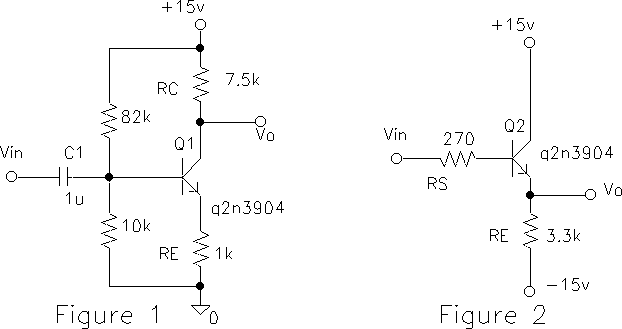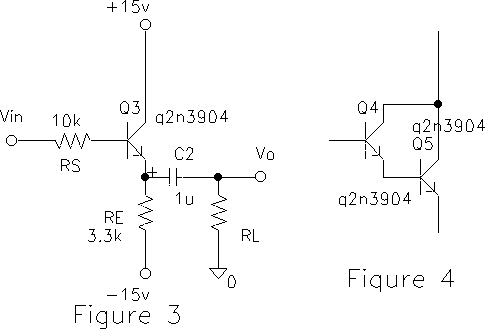EE 321 Lab
Lab 9: BIPOLAR JUNCTION TRANSISTORS, Part II
Prelab for Lab 9

In this lab we will In this lab we will investigate a bipolar junction transistor (BJT) amplifier and emitter follower.

The amplifier circuit of last week's lab is impractical because the bias or operating point depends on the beta of the transistor. The following bias technique controls the voltage across RE and hence the emitter current IE (Example 4.7).

1. Construct the circuit in Figure 1 and measure the d.c. bias voltages at the base, emitter and collector. From your measurements, determine VBE, VBC. Is the transistor biased in its active mode? What are the d.c. (Q `quiescent') values of IC, IE?2) Apply a triangle wave to the input and measure the voltage gain. Is the gain close to that found in the prelab?

3) The voltage gain can be increased by decreasing RE. Reduce RE to zero for signal frequencies by `bypassing' it with a large capacitor (100 uF) in parallel with RE. (Be sure to observe the correct polarity of the electrolytic capacitor.) Reduce the input amplitude and look at the output waveform.

Further reduce the input amplitude until the output is approximately linear and measure the gain. The gain has been increased to the large values, but at what expense?

The Emitter Follower Amplifier

4) When the input signal is applied to the base and the output is taken at the emitter, Figuure 2, the amplifier is called an `emitter follower'. This is because the emitter voltage `follows' the base voltage. Emitter follower amplifiers are useful because they have a high input impedance and a low output impedance. They amplify the signal power by increasing its current, and are therefore sometimes called a `current booster'.

Construct the circuit in Figure 2.

• With zero (ground input) or small input signal, measure and note the d.c. bias voltages.
• With an input, measure the voltage gain (magnitude and sign).
• The dynamic range of the output is the largest that it can be without saturating or cliping. Measure the dynamic range of the output voltage (decrease the supply voltages to +-12 and measure both vout limits). What causes the output to limit in each case (when is the transistor saturated, active, cutoff)?
• What would happen to vout if the emitter resistor were connected to ground instead of -15V? Try it. What does this mean.
5) Measure Zin at the base of the transistor in the following way.
• Increase the source resistance RS until vout is reduced by about 1/2 (or by some other value).
• Sketch the equivalent circuit (use an equivalent circuit of an amplifier from Ch 1, not the transistor model) and compute Zin from your measurement.
• From the theoretical result that Zin = ( beta + 1) [re + RE ] and re=alpha/gm, estimate the beta of your transistor.
6) Simulate a signal of 10 k Ohms source resistance by setting RS = 10 k Ohms (see Figure 3). Measure the output impedance Zout by loading the output with about 220 Ohms resistance. Use a d.c. blocking capacitor as shown to prevent the load from changing the bias voltages. Ensure that the capacitive impedance is small compared to 220 Ohms by working at a signal frequency of about 10 kHz. Decrease the input until the output is not clipped.Sketch an equivalent circuit for the amplifier output and compute Zout from your measurement. Compare with the theoretical value Zout = [re + RS /( beta + 1)] || RE. How much output voltage would you have gotten by connecting RL directly to the source (RS is part of the source)? What does this show?

7) EXTRA CREDIT. In the emitter follower (Figure 3) replace the transistor by two transistors connected in a Darlington configuration, (Figure 4): What is the effective beta of this `super beta' transistor?   Measure Zin and Zout of the follower now?